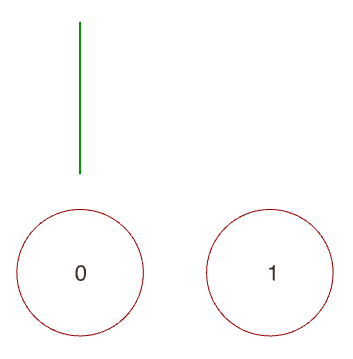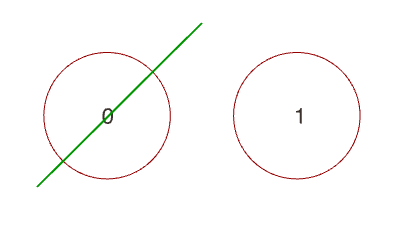# Circle Line Intersection weirdness?

Hi,

What’s the logic behind letting lines intersect what seems like each and every circle in a scene?

For instance, the line in green intersects both circles 0 and 1, although it should only intersect circle 0 when interpreted as an infinite line.However, it finds an intersection with circle 1 as well (cf. GH panel)?

The same goes for “physical” line circle intersections?Here’s my script:

``````import Rhino.Geometry as rg

for i in range(len(C)):
rc, t1, _, t2, _ = rg.Intersect.Intersection.LineCircle(L, C[i])
print "Circle", i, ":", t1, t2, rc
``````

Is this a bug?

Dear @diff-arch
looks like a bug to me.
the Intersection returns the closest point from the circle to the line.

workarround:

``````import Rhino.Geometry as rg

pts = []
rc, t1, p1, t2, p2 = rg.Intersect.Intersection.LineCircle(L, C)
if int(rc) > 0 and (L.DistanceTo(p1,False) < 0.001):
pts.append(p1)
if int(rc) > 1 and (L.DistanceTo(p2,False) < 0.001):
pts.append(p2)
print "Circle", ":", t1, t2, rc, bool(p1), bool(p2)
``````

( i changed to item-Access and added output pts of the scripting component)
you should always check rc:

If Single is returned, only t1 and point1 will have valid values. If Multiple is returned, t2 and point2 will also be filled out.

check the enum “rc” and the distance to the line as workarround

1 Like

Yes, I made a little deep dive into the topic, and it seems that to find an intersection between a circle and line, you can construct a line from the center of the circle perpendicular to the line. If the intersection point of the original and the new line lies within the circle, there’s a two point intersection between the circle and the line and if it’s on the circle there’s a single point intersection.

Thanks.

This should be `pts.append(pi)` in Python.

This is shorter but I like the more explicit version better.

I’m aware of that, but it seems that t2 simply inherits the value of t1 when the intersection is single, and is not not filled out.

the planar / 2d case is described here:

(edit my initial point add → append… sorry i checked if the error is the same (yes) in a c# script)

Here’s my workaround function, inspired by your suggestion.
It’s only meant to check for intersections, nothing more.

``````MTOL = sc.doc.ModelAbsoluteTolerance

def intersecting(line, circle):
relation, t1, pt1, t2, _ = rg.Intersect.Intersection.LineCircle(line, circle)
if relation == rg.Intersect.LineCircleIntersection.Multiple:
if (t1 >= 0.0 and t1 <= 1.0) and (t2 >= 0.0 and t2 <= 1.0):
return True
elif relation == rg.Intersect.LineCircleIntersection.Single:
if line.DistanceTo(pt1, False) < MTOL:
return True
return False
``````

@dale, is this indeed a bug?